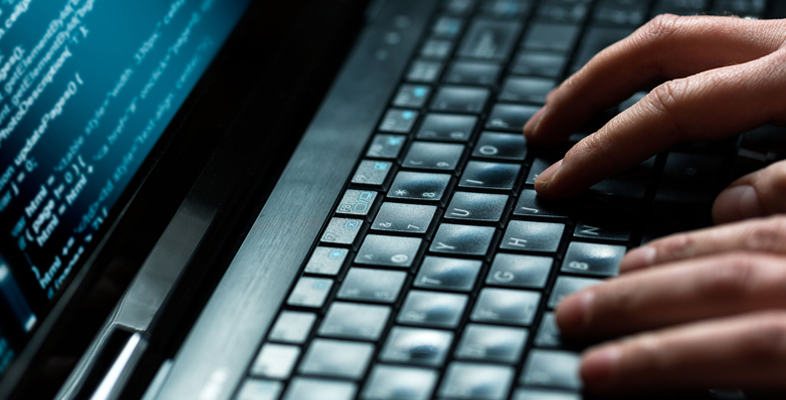Science, Maths & Technology

### Become an OU studentSimple coding

Start this free course now. Just create an account and sign in. Enrol and complete the course for a free statement of participation or digital badge if available.

# 6 Iteration (part 2)

More on iteration and repetition in Python programming language.

Let’s use input to ask for the price of orders instead of fixing them in a list. This requires a new iteration: an infinite loop that keeps asking until the user types ‘stop’, for example. We also need to convert the string returned by input into a number we can add to the expenses. The algorithm is:

1. let the expenses be zero
2. forever repeat the following:
1. let answer be the reply to the question “Price of ordered item?”
2. if the answer is equal to “stop”:
• exit the loop
1. otherwise:
• i.let the price be the answer converted to a decimal number
• ii.add the price to the expenses

Test the code below: provide the same values as before (4.35, 2, 19.95, 22.70 and 5), followed by stop, and check the sum is at the end 54.

Interactive feature not available in single page view (see it in standard view).

In Python, the ‘while condition: block’ instruction keeps executing the block while the condition is true. In this case the condition is always True (note the initial uppercase), which leads to an infinite loop. Doing forever the same thing can get a bit tedious, so at some point I have to break free with the appropriately named break instruction. At that point the computer starts executing whatever code follows the loop.

Note that food and drink prices may be in pence or cents, so I need to convert the user’s input string to a decimal number (called a floating point number in Python), using the function float instead of int.

Note also that to check if two values or variables are equal, we write two equal signs, because a single equal sign is the assignment instruction.

## Activity 10

Put a complete program together, that starts as above, then asks the user for the number of people in the group, computes the tip and the VAT (20% on the expenses) and finally prints the total bill.